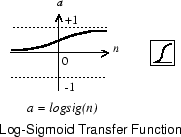# logsig

Log-sigmoid transfer function

## Syntax

``A = logsig(N)``
``dA_dN = logsig('dn',N,A,FP)``
``info = logsig(code)``

## Description

example

``` TipTo use a logistic sigmoid activation for deep learning, use `sigmoidLayer` or the `dlarray` method sigmoid. `A = logsig(N)` takes a matrix of net input vectors, `N` and returns the `S`-by-`Q` matrix, `A`, of the elements of `N` squashed into `[0, 1]`.`logsig` is a transfer function. Transfer functions calculate a layer’s output from its net input.```
````dA_dN = logsig('dn',N,A,FP)` returns the `S`-by-`Q` derivative of `A` with respect to `N`. If `A` or` FP` are not supplied or are set to `[]`, `FP` reverts to the default parameters, and `A` is calculated from `N`.```
````info = logsig(code)` returns information about this function. For more information, see the code argument description.```

## Examples

collapse all

This example shows how to calculate and plot the log-sigmoid transfer function of an input matrix.

Create the input matrix, `n`. Then call the `logsig` function and plot the results.

```n = -5:0.1:5; a = logsig(n); plot(n,a) ```

Assign this transfer function to layer `i` of a network.

```net.layers{i}.transferFcn = 'logsig'; ```

## Input Arguments

collapse all

Net input column vectors, specified as an `S`-by-`Q` matrix.

Information you want to retrieve from the function, specified as one of the following:

• `'name'` returns the name of this function.

• `'output'` returns the `[min max]` output range.

• `'active'` returns the `[min max]` active input range.

• `'fullderiv'` returns 1 or 0, depending on whether `dA_dN` is `S`-by-`S`-by-`Q` or `S`-by-`Q`.

• `'fpnames'` returns the names of the function parameters.

• `'fpdefaults'` returns the default function parameters.

## Output Arguments

collapse all

Output vectors, returned as an `S`-by-`Q` matrix, where each element of `N` is squashed from the interval ```[-inf inf]``` to the interval `[0 1]` with an "S-shaped" function.

## Algorithms

`logsig(n) = 1 / (1 + exp(-n))`

## Version History

Introduced before R2006a